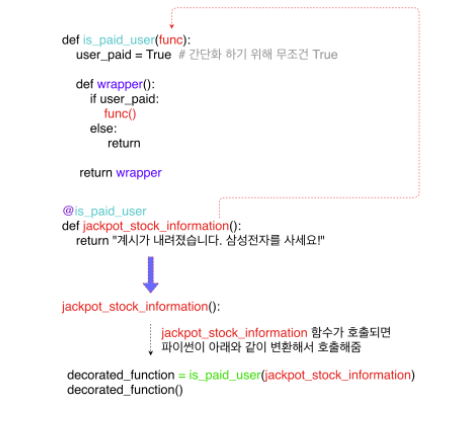# TIL: Nested Functions

markkimjr·2021년 1월 17일
0Like nested lists, nested dictionaries, etc., FUNCTIONS can also be nested!!!def parent_function():
def child_function():
print("this is a child function")

child_function()

parent_function()

> "this is a child function"

### Why use nested functions?

If there are lines of repetitive code, using nested functions is a good way to group and organize these lines of code in a more logical and easy-to-read manner.

2. Closure
Nested functions are used to enclose the parent function's variable/information and use/refine the enclosed variable/information in a different way. For nested functions to work, the parent function must return the nested function. This means that the nested function cannot be called outside in the global frame and can only be accessed by calling the parent function (thus, a nested function). This is especially useful when there is a certain function that you want to use, but you also want to impose restrictions that store the nested information in the function away until it is time to actually use it

For example...

def generate_power(base_number):
def nth_power(power):
return base_number ** power
return nth_power

calculate_power_of_two = generate_power(2)

calculate_power_of_two(7)
> 128

calculate_power_of_two(10)
> 1024

calculate_power_of_seven = generate_power(7)

calculate_power_of_seven(3)
> 343

calculate_power_of_seven(5)
> 16907

In the above example, the base_number (2) is initially stored away in the nested function nth_power(power), which is returned when generate_power(2) is called. nth_power(power) is assigned to the variable calculate_power_of_two, and when calculate_power_of_two(8) is called, the initial base_number (2) is used to complete the operation: base_number ** 7. This value is then returned by the nested function (nth_power(power)). This same process occurs when calculate_power_of_two(10) is called.

#### @Decorators

A decorator is a function that contains a nested function and that takes another function as its parameter:

def is_paid_user(func):
user_paid = True # 간단화 하기 위해 무조건 True

def wrapper(*args, **kwargs):
if user_paid:
func()
else:
return

return wrapper

@is_paid_user
def jackpot_stock_information():
return "Samsung is a hot stock right now. Invest right away!"

In the above code, @is_paid_user decorates the function "jackpot_stock_information()". The function, is_paid_user(func), is called first, and it takes jackpot_stock_information() as its parameter when jackpot_stock_information() is called.